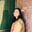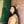Trusted answers to developer questions
Trusted Answers to Developer Questions

Related Tags

numpy
python

# What is numpy.lcm() in Python?Umme Ammara

Grokking Modern System Design Interview for Engineers & Managers

Ace your System Design Interview and take your career to the next level. Learn to handle the design of applications like Netflix, Quora, Facebook, Uber, and many more in a 45-min interview. Learn the RESHADED framework for architecting web-scale applications by determining requirements, constraints, and assumptions before diving into a step-by-step design process.

The Least Common Multiple (LCM) is the smallest number that is the multiple of all the numbers in question. Python’s numpy.lcm() method allows us to calculate the LCM of numbers and arrays.

## Syntax

numpy.lcm() is declared as follows:

numpy.lcm(x1, x2, /, out=None, *, where=True, casting='same_kind', order='K', dtype=None, subok=True[, signature, extobj]) = <ufunc 'lcm'>


In the syntax above, x1 and x2 are non-optional parameters, and the rest are optional parameters.

A universal function (ufunc) is a function that operates on ndarrays in an element-by-element fashion.

## Parameters

The numpy.lcm() method takes the following compulsory parameters:

• x1 and x2 [array-like, int] - array of integer values. If the shapethe shape of an array is the number of elements in each dimension of x1 and x2 is different, they must be broadcastable to a common shape for representing the output.

The numpy.lcm() method takes the following optional parameters:

 Parameter Description out represents the location into which the output of the method is stored. where True value indicates that a universal function should be calculated at this position. casting controls the type of datacasting that should occur. The same_kind option indicates that safe casting or casting within the same kind should take place. order controls the memory layout order of the output function. The option K means reading the elements in the order they occur in memory. dtype represents the desired data type of the array. subok decides if subclasses should be made or not. If True, subclasses will be passed through.

## Return value

numpy.lcm() returns the lowest common multiple of the absolute values of the input. The return type is either ndarray or scalar, depending on the input type.

## Examples

The examples below show the different ways numpy.lcm() is used in Python.

### LCM of two numbers

The code below outputs the least common multiple of two numbers, 12 and 42. The result is shown below:

import numpy as np a = 12b = 42result = np.lcm(a,b)print(result)

### LCM of an array

To find the LCM of all values in a given array, the reduce() method is used. In the code below, the reduce method utilizes the lcm method on each array element and reduces the array by one dimension. The result is shown in the code snippet below:

import numpy as np arr = np.array([3,12,15])result = np.lcm.reduce(arr)print(result)

### LCM of two arrays

numpy.lcm() calculates the least common multiple of the elements in the same location of the two arrays and returns an array.

In the example below, the first element of arr1 is 12, and the first element of arr2 is 20. The LCM of 12 and 20 is 60. Hence, the result array has 60 as its first element. This is shown in the example here:

import numpy as nparr1 = np.array([12,8,4])arr2 = np.array([20,16,2])result = np.lcm(arr1,arr2)print(result)

RELATED TAGS

numpy
python

CONTRIBUTORUmme Ammara